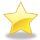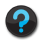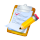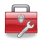# Using Statistical Regression Methods in Education Research# Module 5 - Ordinal Regression

### Objectives

1. Understand the principles and theories underlying Ordinal Regression
2. Understand the assumption of Proportional Odds and how to test it
3. Be able to implement Ordinal Regression analyses using SPSS and accurately interpret the output
4. Be able to include interaction terms in your Ordinal Regression model and to accurately interpret the output
5. Appreciate the applications of Ordinal Regression in education research and think about how it may be useful in your own research## Start Module 5: Ordinal Regression###### Using multiple variables to predict ordinal outcomes.You can jump to specific pages using the contents list below. If you are new to this module start at the Introduction and work through section by section using the 'Next' and 'Previous' buttons at the top and bottom of each page. Be sure to tackle the exercise and the quiz to get a good understanding.

This Module is also available as a downloadable PDF for those of you who prefer to read from a page. You do miss out on the glossary and some of the site's more interactive features though... Module 5: Ordinal RegressionContents

5.1 Introduction

5.2 Working with ordinal outcomes

5.3 Key assumptions of ordinal regression

5.5 Teacher expectations and tiering

5.6 Example 2 - Running an ordinal regression for mathematics tier of entry

5.7 Example 3 - Evaluating interaction effects in ordinal regression

5.8 Example 4 - Including a control for prior attainment

5.9 What to do if the assumption of proportional odds is not met?

5.10 Reporting the results of ordinal regression

5.11 Conclusions

Quiz

Exercise

 HomeModulesSite GuideModule 5 ContentsResources